## Abstract

In this paper, the effects of different widths and lengths of collar around bridge abutment on local scour depth are studied numerically and experimentally. Numerical simulation of scour hole evolution at bridge abutment is more convenient than the experimental modeling, because the computational cost and time have significantly decreased. The numerical model solves 3-D Navier–Stokes equations and bed load conservation equation. The kε turbulence model is used to solve the Reynolds-stress term. The simulated results are verified using the laboratory experiments. In addition, the multiple linear regressions are applied to correlate the maximum local scour depth with the other independent parameters. It was found that the relative length of collar 0.73 around bridge abutment reduces the maximum scour depth by 69% compared to no-collar case. Moreover, the results of 3-D numerical model and regression models agree well with the experimental data.

## Keywords

Numerical models; Collar; Bridge abutments; Local scour; SSIIM

## Nomenclature

D50- the median sand size

σg- the geometric standard deviation

U- mean flow velocity

Uc- mean approach velocity at the threshold condition

d- sediment particle diameter

τ- bed shear stress

τc- critical bed shear stress

ρw- density of water

ρs- density of sediment

ν- kinematic viscosity

g- gravitational acceleration

K- von Karmen constant

U- shear velocity

z- height above the bed

yt- tail water depth

Ft- tail Froude number

ds- maximum local scour depth

L1- collar width

b- abutment width in lateral direction

Lc- length of collar in longitudinal direction

L- length of bridge abutment

## 1. Introduction

Scour around bridge piers and abutments is considered one of the most important fields of hydraulic researches. The scour phenomenon may endanger the whole structure after long or short run depending upon the extent of scouring processes. Richardson and Abed  quoted a study produced in 1973 for the U.S. Federal Highway Administration, that concluded of 383 bridge failures, 25% involved pier damage and 72% involved abutment damage; so many researchers investigated the scour phenomenon at bridge foundations, i.e. bridge piers and abutments. The local scour depth around spur dikes and bridge abutments was estimated in alluvial rivers . Static reliability model was developed for the assessment of local scouring reliability around bridge abutments . Overview of scour types and scour-estimation difficulties faced at bridge abutments was investigated , and clear-water scour development at bridge abutments was presented . Numerical simulation of scour depth evolution around bridge piers was investigated ,  and . Scour around bridge abutment was studied experimentally , , , ,  and . Time-wise variation of scouring at bridge abutments was studied . Numerical models were also presented to simulate the scour depth around bridge abutment  and . Group method of data handling (GMDH) network was used to predict abutments scour depth of the bridges . Gene expression programming and artificial neural networks were used to predict the time variation of scour depth at a short abutment . In this study, the local scour around bridge abutment was studied experimentally and numerically. The effect of different widths and lengths of collar at abutment edge, on local scour depth were investigated. These collars were considered as a tool that maximize the reduction of maximum local scour around bridge foundation and hence ensure greater safety of the structure against the harmful scour around bridge foundation, and hence longer life of the hydraulic structure is expected. The numerical models were created by using sediment simulation in water intakes with multiblock option (SSIIM) program. This 3D CFD model was based on the finite volume method to solve the Navier–Stokes equations .

## 2. Experimental work

The experimental work was carried out in a re-circulating channel with 5.7 m length, 20 cm depth and 40 cm width (Fig. 1 and Photo 1). Stones with different sizes were used at entrance to damp carefully disturbances. The flume entrance and outlet lengths are 0.3 and 1.4 m, respectively. The discharge was measured using a pre-calibrated orifice meter. The median sand size (D50) is 1.77 mm. The sediment is to be considered as uniform at which the geometric standard deviation of the particle size distribution is less than 1.3 (${\textstyle {\sigma }_{g}=D_{84}/D_{50}=1.29}$). Sieve analysis of movable bed is shown in Fig. 2. The experimental work was conducted under the clear-water condition. Clear water scour occurs for velocities up to the threshold for the general bed movement, i.e., U/Uc ⩽ 1 (U, is the approach flow velocity, and Uc, is mean approach velocity at the threshold condition), . In the present study the value of U/Uc equals 0.86 (i.e., clear water scour). For each test of the experimental program, the sand soil with thickness 10 cm, was leveled along the entire length of flume using a wooden screed with the same width as the flume. The sand level was checked randomly in points with a point gauge. The flume was slowly filled with water to the required depth. The pump was then turned on and its speed increased slowly until the desired flow rate was achieved, after that the tailgate was adjusted to get the required water depth. At the end of the test the pump was turned off and the flume was drained slowly without disturbing the scour topography. The bed topography was measured with point gauge with 0.01 mm accuracy on a grid with meshes of 3 cm × 3 cm (sometimes 1 cm × 1 cm depending on the bed topography) over an area of 2.5 m × 0.4 m spanning between 1.0 m upstream and 1.1 m downstream from the abutment. The grid pattern was dense to obtain accurate bed topography at the end of each experiment. Wooden edge abutments with 40 cm length and 7.5 cm widths were installed in the channel sides. The used protective plate (collar) in the experiments is 2 mm thickness and is made from Perspex. The collars fixed at the same level as the mobile bed. The collar widths are 4.5, 6 and 7.5 cm. The lengths of collar are 12, 13.5, 15, 20.5, 29, 37.5, 46 and 52 cm, Table 1, (Photo 2). The total number of experiments is 90. Details of the experimental conditions are summarized in Table 1. Fig. 3 presents the required time for each test, in which the relationship between ds/ds-Equilibrium was plotted against the time. It was found that 90% of maximum scour depth was achieved at 2 h.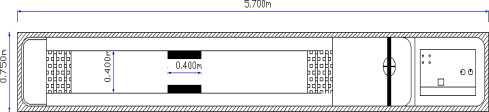Figure 1. Definition sketch of a re-circulating flume.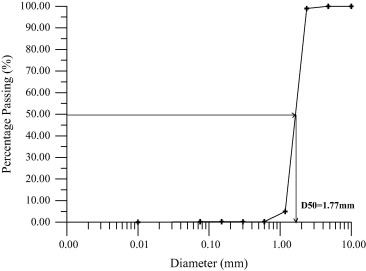Figure 2. Sieve analysis of movable bed soil.

 Discharge (l/s) 3.5 Median sand size (mm) 1.77 Abutment width (b) cm 3.75, 5.0, and 7.5 Flow depth (cm) 3–7 Collar width (L1) cm 4.5, 6 and 7.5 Froude number 0.20–0.55 Collar length (Lc) cm 12, 13.5, 15, 20.5, 29, 37.5, 46 and 52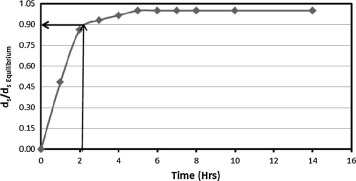Figure 3. Ratio of maximum to equilibrium scour depths (ds/dsEquilibrium) versus time.

## 3. The numerical model

The SSIIM program solves the Navier–Stokes equations with the kε on a three dimensional and general non-orthogonal coordinates. These equations are discretized with a control volume approach. An implicit solver is used, producing the velocity field in geometry. The velocities are used when solving the convection–diffusion equations. The Navier–Stokes equations for non-compressible and constant density flow can be modeled as follows:

 ${\frac {\partial u_{i}}{\partial t}}+U_{j}{\frac {\partial u_{i}}{\partial x_{j}}}=$${\frac {1}{\rho }}{\frac {\partial }{\partial x_{j}}}\left[-\right.$$\left.p{\delta }_{ij}-\rho {\overline {u_{i}}}{\overline {u_{j}}}\right]$
(1)

The left term on the left side of the Eq. (1) indicates the time variations. The next term is the convective term. The first term on the right-hand side is the pressure term and the second term on the right side of the equation is the Reynolds stress. The Reynolds stress is evaluated using turbulence model kε. The free surface is calculated using a fixed-lid approach, with zero gradients for all variables. The locations of the fixed lid and its movement are as a function of time and water flow field are computed by different algorithms. The 1D backwater computation is the default algorithm and it is invoked automatically. Formula developed by Van Rijn  was used to calculate the equilibrium sediment concentration close to the bed. This equation has the following form:

 $C_{\mbox{bed}}=0.015{\frac {d^{0.3}{\left[(\tau -{\tau }_{c})/{\tau }_{c}\right]}^{1.5}}{a{\left[\left(({\rho }_{s}-{\rho }_{w})g)/({\rho }_{w}{\nu }^{2}\right)\right]}^{0.1}}}$
(2)

where Cbed is the sediment concentration, d is the sediment particle diameter, a is a reference level set equal to the roughness height, τ is the bed shear stress, τc is the critical bed shear stress for movement of sediment particles according to Shield’s curve, ρw and ρs are the density of water and sediment respectively, ν is the Kinematic viscosity of the water and g is the gravitational acceleration.

Van Rijn’s formula  is used to calculate the bed load discharge (qb), and the equation has the following form:

 ${\frac {q_{b}}{D_{50}^{1.5}{\sqrt {\left(({\rho }_{s}-{\rho }_{w})g\right)/{\rho }_{w}}}}}=$$0.053{\frac {d^{0.3}{\left[(\tau -{\tau }_{c})/{\tau }_{c}\right]}^{1.5}}{D_{50}^{0.3}{\left[\left(({\rho }_{s}-{\rho }_{w})g\right)/({\rho }_{w}{\nu }^{2})\right]}^{0.1}}}$
(3)

The influence of rough boundaries on fluid dynamics is modeled through the inclusion of the wall law:

 ${\frac {U}{U_{_{\ast }}}}={\frac {1}{K}}ln(30z/K_{s})$
(4)

where ks equals to the roughness height, K   is von Karmen constant, ${\textstyle U}$ is the mean velocity, ${\textstyle U_{_{\ast }}}$ is the shear velocity and z is the height above the bed.

## 4. Model geometry and properties

A structured grid mesh on the xyz plane was generated, a three dimensional grid mesh with 234 elements in the x-direction, 66 elements in the y-direction and 22 elements in the z-direction. An uneven distribution of grid lines in both horizontal and vertical directions was chosen in order to keep the total number of cells in an acceptable range and to get valuable results in the area. The following grid line distributions were chosen: In X-direction: 3 cells with a 0.25 m, 10 cells with a 0.05 m, 25 cells with a 0.02 m, 160 cells with a 0.005 m, 20 cells with a 0.02 m, 10 cells with a 0.05 m and 5 cells with a 0.11 m respectively. In Y-direction: 30 cells with a 0.005 m, 5 cells with a 0.02 m and 30 cells with a 0.005 m respectively. In Z-direction: 10 cells with 1% height of the water depth, 4 cells with 5% of the water depth and 7 cells with 10% of the water depth. The Abutment was generated by specifying its ordinates, and then the grid interpolated using the elliptic grid generation method. However, the Abutment was generated by blocking the area of the Abutment.

## 5. Analysis and discussion

### 5.1. Effect of relative collar width at abutment (L1 − b)/b)

The effect of different widths of collar at abutment edge on local scour depth was investigated experimentally and numerically for contraction ratio of abutment equal to 0.37. The experimental data for the no-collar case and other data  and  are plotted in Fig. 4. In addition, the relationship between the maximum relative scour depth around bridge abutment (ds/yt, where ds is the maximum local scour depth, and yt is the tail water depth) and the tail Froude number (Ft) is presented in the same previous figure, for different relative collar widths of bridge abutment (L1  b)/b = 0.6, 0.8 and 1.0, where L1 is the collar width, and b is the abutment width in lateral direction. From this figure, it can be concluded that as the relative collar width increases, the relative scour depth decreases and vice versa. The increasing of collar width shares to absorb the downward velocity and prevent it to reach the mobile bed. This leads to high protection for the bridge abutment. The scour contour maps for different relative widths of collar ((L1 − b)/b = 0.6, 0.8 and 1.0) are presented in Fig. 5. It is clear that the scour hole dimensions are small for the largest relative collar width. The maximum local scour depth was reduced by 31%, 37% and 44% by using relative collar widths 0.6, 0.8, and 1.0, respectively compared to no collar case. The velocities’ distribution and bed surface profile at lateral cross-sections are presented in Fig. 6. This figure shows that the scour dimensions and velocity fields for the no-collar case are larger compared to the other collar cases, i.e. (L1  b)/b = 0.6, and 1.0. Also, the bed surface profiles for the experimental and simulated cases are near together. The scour contour maps for a typical case created by the simulated and experimental models are presented in Fig. 7. In addition, the simulated values of local scour depth created by the 3D-computational fluid dynamic model were compared to the measured data in Fig. 8. It was found that the simulated models produce scour depths close to the experimental values with correlation coefficient R2 = 97% and standard error 2.5%.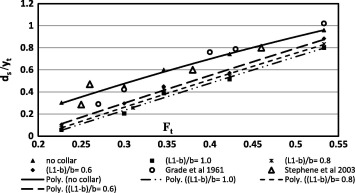Figure 4. The relationship between ds/yt and Ft for different collar widths (L1 − b)/b.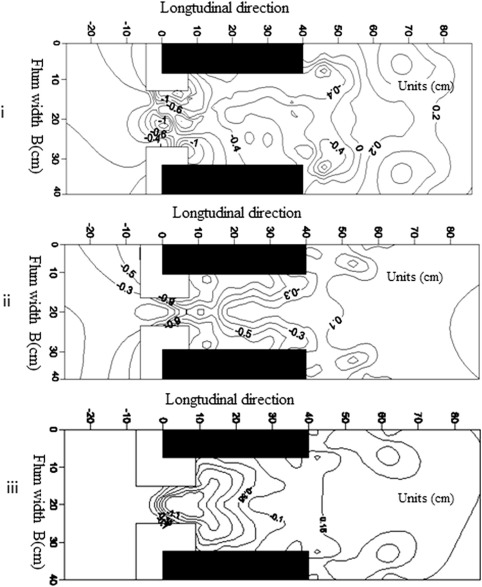Figure 5. Scour contour maps for (i) (L1 − b)/b = 0.6, (ii) (L1 − b)/b = 0.8 and (iii) (L1 − b)/b = 1.0 at Ft = 0.35.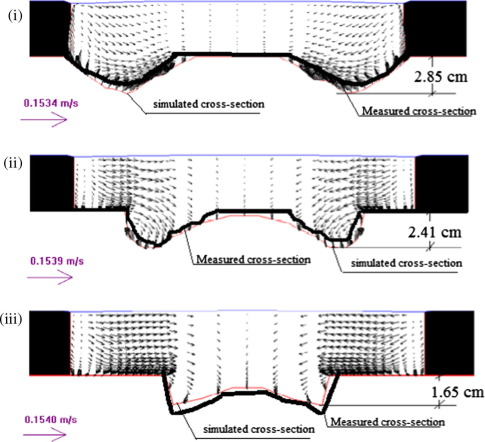Figure 6. Velocity distribution for Lateral cross-section near bridge abutment for (i) no collar, (ii) (L1 − b)/b = 0.6, and (iii) (L1 − b)/b = 1.0 at Ft = 0.42.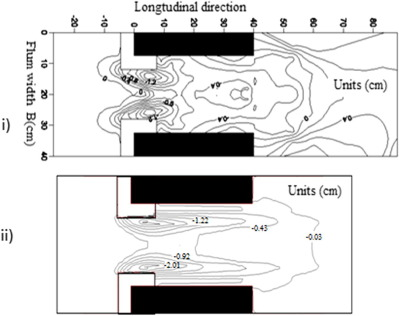Figure 7. Scour contour maps experimentally and numerically for (i) (L1 − b)/b = 0.6 and at Ft = 0.42.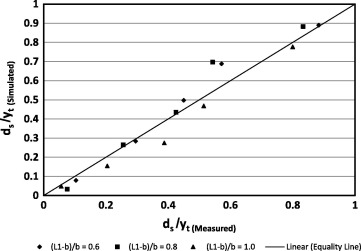Figure 8. The simulated versus experimental values for Q = 3.47 l/s and different widths of collar (L1 − b)/b.

### 5.2. Effect of relative collar length at abutment (Lc/L) on local scour depth

The effect of different lengths of collar around bridge abutment on local scour depth was investigated experimentally and numerically at relative width of collar equal to 0.8 and contraction ratio of abutment equals 0.37. The relationship between the maximum relative scour depth around bridge abutment (ds/yt) and the tail Froude number (Ft) is shown in Figure 9 and Figure 10 for different relative collar lengths around bridge abutment (Lc/L = 0.34, 0.51, 0.73, 0.94, 1.15 and 1.3, where Lc is length of collar in longitudinal direction, and L, is the length of bridge abutment). From these figures, the maximum local scour depth is reduced by 38%, 56%, 69%, 62%, 65%, and 59% for the previous relative collar lengths respectively. The relative length of collar equals 0.73 produces minimum scour depth compared to the other relative lengths of collar around the bridge abutment. The scour contour maps, clarifying the shape of scour distributions for different relative lengths of collar around bridge abutment, are presented in Fig. 11. It was found that relative lengths of collar from 0.73 to 1.15 around abutment have smaller scour dimensions compared to the other ones.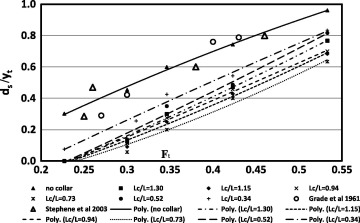Figure 9. The relationship between ds/yt and Ft at different relative lengths of collar Lc/L.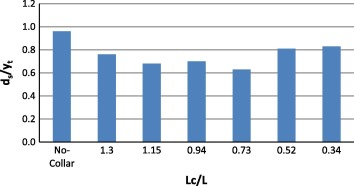Figure 10. The relationship between ds/yt and Lc/L at Ft = 0.53.

Figure 11.

Scour contour maps for (i) Lc/L = 0.34, (ii) Lc/L = 0.51 and (iii) Lc/L = 0.73 at Ft = 0.42, (iv) Lc/L = 0.94, (v) Lc/L = 1.15 and (vi) Lc/L = 1.30 at Ft = 0.42.

The combination between the smooth surface for collar and the mobile bed for this range of collar lengths is considered as a resistance to flow field and may be affected positively for the decreasing local scour depth at bridge abutment. For the shorter collar length the protective area is not enough to have small values of scour depth. On the other hand, for longer lengths of the collar, the smooth surface of collar may be affected negatively on the velocity distribution (Fig. 12ii). The bed surface profiles for experimental and simulated models seem to be close together, Fig. 12. The simulated values of local scour depth created by the 3D computational fluid dynamic model are compared to the measured data in Fig. 13. It is clear that the simulation model expresses well the experimental models, at which the correlation coefficient and standard error are 94% and 5.5%, respectively.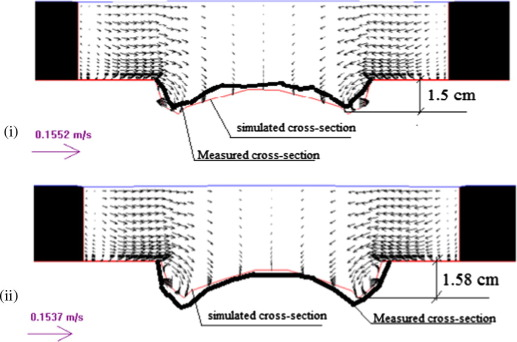Figure 12. Velocity distribution for Lateral cross-section (near bridge abutment) for (i) Lc/L = 0.73 and (ii) Lc/L = 1.3.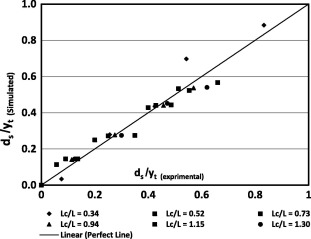Figure 13. The numerical versus experimental values for Q = 3.47 l/s and different lengths of collar (Lc/L).

### 5.3. Statistical analysis

Multiple linear regressions were applied for all simulated cases to have statistical prediction models correlating the maximum relative scour depth with other independent parameters. Many of trials were carried out to have a general equation representing the whole independent parameters as follows:

 ${\frac {d_{s}}{y_{t}}}=-0.23+2.18\quad F_{t}-0.14{\frac {L_{1}-b}{b}}-$$0.17{\frac {L_{c}}{L}}$
(5)

The correlation coefficients and stander errors of Eq. (5) are 91%, and 7% respectively. Fig. 14 presents the predicted values of relative scour depth versus measured data sets. It was found that the predicted equation expresses well the measured data.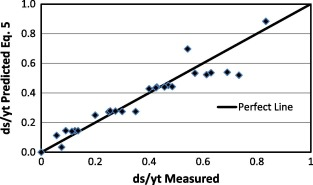Figure 14. Predicted values of Eq. (5) versus measured data for relative scour depth.

Photo 1.

Re-circulating flume.

Photo 2.

The protective plate.

## 6. Conclusions

The present study focuses on experimental and numerical simulation of scour at bridge abutment in sand soil. Different collar widths and lengths at bridge abutment are examined experimentally and by employing a 3D numerical model (SSIIM program). The maximum relative scour depth decreases as the relative collar width increases and vice versa. In addition, the relative length of collar equals 0.73 around bridge abutment produces minimum scour depth compared to the other relative lengths of collars. In addition, the simulated results show the ability of SSIIM for modeling the local scouring at bridge abutments having different collar widths and lengths with average correlation coefficient of 95%. The predicted equation for relative scour depth using multiple linear regressions agrees well with the measured data.

### Document informationPublished on 12/04/17

Licence: Other

### Document Score0

Views 26
Recommendations 0